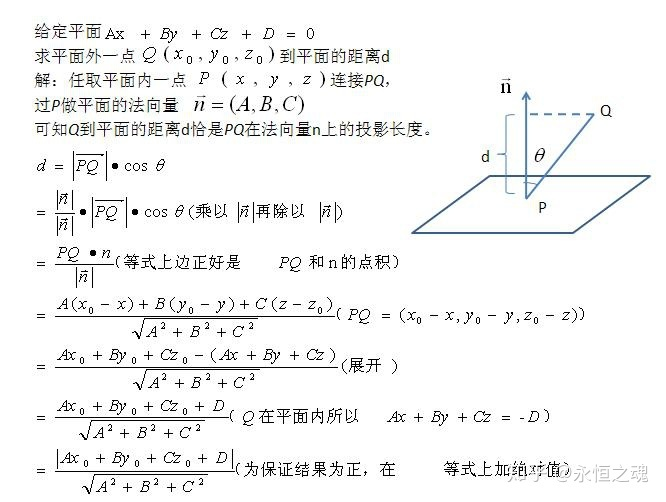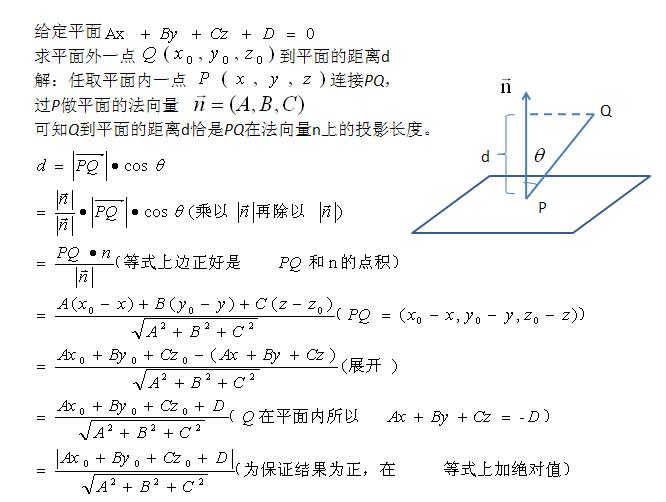• 最近在复习SVM的知识，其中有一个点到超平面的公式无法理解，看了原博主的博文感觉豁然开朗，故在这里收藏一下，...点到平面的距离公式 准备知识 平面的一般式方程 Ax +By +Cz + D = 0 其中n = (A, B, C)是

最近在复习SVM的知识，其中有一个点到超平面的公式无法理解，看了原博主的博文感觉豁然开朗，故在这里收藏一下，转自http://www.cnblogs.com/graphics/archive/2010/07/10/1774809.html

点到平面的距离公式

准备知识

平面的一般式方程
Ax +By +Cz + D = 0
其中n = (A, B, C)是平面的法向量，D是将平面平移到坐标原点所需距离（所以D=0时，平面过原点）

向量的模（长度）
给定一个向量V（x, y, z),则|V| = sqrt(x * x + y * y + z * z)

向量的点积（内积）
给定两个向量V1(x1, y1, z1)和V2(x2, y2, z2)则他们的内积是
V1V2 = x1x2 + y1y2 + z1z2

点到平面的距离
有了上面的准备知识，则求点到直线的距离不再是难事，有图有真相如果法相量是单位向量的话，那么分母为1

ORGE中的实现
顺便看一下Ogre中的实现

Real Plane::getDistance (const Vector3& rkPoint) const
{
return normal.dotProduct(rkPoint) + d;
}

可见Ogre默认法相量为单位向量，且没有对结果取绝对值，根据注释，当点与法相量的方向在同一侧时，结果为正，否则为负。

作者：zdd
出处：http://www.cnblogs.com/graphics/

本文版权归作者和博客园共有，欢迎转载，但未经作者同意必须保留此段声明，且在文章页面明显位置给出原文连接，否则保留追究法律责任的权利.


展开全文• 点到平面的距离公式 准备知识 平面的一般式方程 Ax +By +Cz + D = 0 其中n = (A, B, C)是平面的法向量，D是将平面平移到坐标原点所需距离（所以D=0时，平面过原点） 向量的模（长度） 给定一个向量V（x, y, z)...
点到平面的距离公式

准备知识

平面的一般式方程

Ax +By +Cz + D = 0

其中n = (A, B, C)是平面的法向量，D是将平面平移到坐标原点所需距离（所以D=0时，平面过原点）

向量的模（长度）

给定一个向量V（x, y, z),则|V| = sqrt(x * x + y * y + z * z)

向量的点积（内积）

给定两个向量V1(x1, y1, z1)和V2(x2, y2, z2)则他们的内积是

V1V2 = x1x2 + y1y2 + z1z2

点到平面的距离

有了上面的准备知识，则求点到直线的距离不再是难事，有图有真相如果法相量是单位向量的话，那么分母为1

转载自：http://www.cnblogs.com/graphics/archive/2010/07/10/1774809.html

展开全文推导 公式
• SVM算法基础之点到平面的距离公式推导 SVM算法基础之点到平面的距离公式推导 平面的一般式方程 Ax +By +Cz + D = 0 其中n = (A, B, C)是平面的法向量，D是将平面平移到坐标原点所需距离（所以D=0时，平面过原点） ...
SVM算法基础之点到平面的距离公式推导
SVM算法基础之点到平面的距离公式推导
平面的一般式方程
Ax +By +Cz + D = 0
其中n = (A, B, C)是平面的法向量，D是将平面平移到坐标原点所需距离（所以D=0时，平面过原点）
向量的模（长度）
给定一个向量V（x, y, z),则|V| = sqrt(x * x + y * y + z * z)
向量的点积（内积）
给定两个向量V1(x1, y1, z1)和V2(x2, y2, z2)则他们的内积是
V1V2 = x1x2 + y1y2 + z1z2
点到平面的距离
有了上面的准备知识，则求点到直线的距离不再是难事，有图有真相如果法相量是单位向量的话，那么分母为1
ORGE中的实现
顺便看一下Ogre中的实现
Real Plane::getDistance (const Vector3& rkPoint) const
{
return normal.dotProduct(rkPoint) + d;
}
可见Ogre默认法相量为单位向量，且没有对结果取绝对值，根据注释，当点与法相量的方向在同一侧时，结果为正，否则为负。


展开全文机器学习
• 点到平面的距离公式 准备知识 平面的一般式方程 Ax +By +Cz + D = 0 其中n = (A, B, C)是平面的法向量，D是将平面平移到坐标原点所需距离（所以D=0时，平面过原点） 向量的模（长度） 给定一个向量V...

点到平面的距离公式

准备知识

平面的一般式方程
Ax +By +Cz + D = 0
其中n = (A, B, C)是平面的法向量，D是将平面平移到坐标原点所需距离（所以D=0时，平面过原点）

向量的模（长度）
给定一个向量V（x, y, z),则|V| = sqrt(x * x + y * y + z * z)

向量的点积（内积）
给定两个向量V1(x1, y1, z1)和V2(x2, y2, z2)则他们的内积是
V1V2 = x1x2 + y1y2 + z1z2

点到平面的距离
有了上面的准备知识，则求点到直线的距离不再是难事，有图有真相如果法相量是单位向量的话，那么分母为1


展开全文• 空间直线方程及两直线夹角 空间直线一般方程 方向向量 直线的点向式（对称式）方程 两直线夹角 两直线相互垂直和平行充分必要条件
• EBF的平面方程可以用斜截式快速写出，OEBF的距离可以用公式快速求出。 所以这一道题目可以快速算出答案
•   点到平面距离公式推导如下图所示，详细信息参见百度百科：https://baike.baidu.com/item/%E7%82%B9%E5%88%B0%E5%B9%B3%E9%9D%A2%E8%B7%9D%E7%A6%BB/10690055?fr=aladdin   该计算方法在C++中实现代码如下：算法 c++
• 准备知识平面的一般式方程Ax +By +Cz + D = 0其中n = (A, B, C)是平面的法向量，D是将平面平移坐标原点所需距离（所以D=0时，平面过原点）向量的模（长度）给定一个向量V（x, y, z),则|V| = sqrt(x * x + y * y + ...
• 其中n = (A, B, C)是平面的法向量，D是将平面平移坐标原点所需距离（所以D=0时，平面过原点） 向量的模（长度） 给定一个向量V（x, y, z),则|V| = sqrt(x * x + y * y + z * z) 向量的积（内积） 给定两个...
• 设x为N维矢量，w为N维系数矢量，y为标量，b为标量系数，则广义的平面可定义为y=w'x+b（式中 ' 表示转置）。距离公式为可以看到，二维空间中点到直线的距离和三维空间中点到平面的距离是上面公式的特例。...
• 在感知机模型中，输入空间中任意一点 平面S的距离：其推导过程如下：
• 转载博客： https://blog.csdn.net/deecheanW/article/details/89057405...